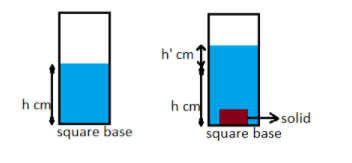QuestionAnswers

# A rectangular container, whose base is a square of side 12 cm, contains sufficient water to submerge a rectangular solid $8{\text{ cm }} \times {\text{ 6 cm }} \times {\text{ 3 cm}}$. Find the rise in the level of water in the container when the solid is in it.$\left( a \right)$ 3 cm$\left( b \right)$ 2 cm$\left( c \right)$ 1 cm$\left( d \right)$ 4 cm

Hint: In this particular questions assume any different variables be the level of the water in the container and the rise in the level of water in the container when the solid is in it, so the increased in the volume of the container when the solid is in it is equal to the volume of solid so use these concepts to reach the solution of the question.Given data:
Rectangular container has a base shape of a square of side 12 cm.
Let the level of the water in the container be h cm.
So the volume of the container is the product of the area of the base and the height of the water level in the container.
Now as we know that the area of the square is side square.
So the area of the square base is = ${\left( {12} \right)^2}$ = 144 square cm.
So the volume of the water in the container is = $A \times h = 144h$ cubic cm.
Now a solid has dimension $8{\text{ cm }} \times {\text{ 6 cm }} \times {\text{ 3 cm}}$completely submerged into the container.
So the volume of the solid is given as, $lbh$ cubic units, where l = length, b = breadth, and h = height of the solid respectively.
So the volume of the solid is = $8 \times 6 \times 3 = 144$ cubic cm.
Let the level of the water in the container increase by h’ cm.
So the total level of the water in the container is equal to (h + h’) cm.
So the total volume of water when the solid is fully submerged in the container = $144\left( {h + h'} \right)$ cubic cm.
So the increase in the volume of water when the solid fully submerged in the container is the difference of the total volume of water when the solid fully submerged in the container and volume of the water in the container.
So the increased in the volume of water when the solid fully submerged in the container is,
$\Rightarrow 144\left( {h + h'} \right) - 144h = 144h'$ Cubic cm.
Now, the increase in the volume of the container when the solid is in it is equal to the volume of solid.
$\Rightarrow 144h' = 144$
$\Rightarrow h' = 1$ Cm.
So the rise in the level of water in the container when the solid is in it is 1 cm.
Hence option (c) is the correct answer.

Note:Whenever we face such types of questions the key concept we have to remember is that the volume of the solid is the product of its length, breadth and height of the solid and always recall that the volume of the rectangular container having square base is the product of the area of the square base and the height of the rectangular container.